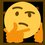# Golden Ratio Version 1

$\frac{a+b}{a}=\frac{a}{b}\overset{\text{def}}{=}\phi$

\begin{aligned} \frac{a+b}{a}&=\frac{a}{b}\\ ab+b^2&=a^2\\ b^2+2\left(\frac{a}{2}\right)b+\left(\frac{a}{2}\right)^2&=a^2+\left(\frac{a}{2}\right)^2\\ \left(b+\frac{a}{2}\right)^2&=a^2+\frac{a^2}{4}\\ b+\frac{a}{2}&=±\sqrt{\frac{5a^2}{4}}\\ b&=\frac{\left(1±\sqrt5\right)a}{2} \end{aligned}

\begin{aligned} \phi&=\frac{a}{b}\\ &=\frac{a}{\frac{\left(1±\sqrt5\right)a}{2}}\\ &=\frac{2a}{1±\sqrt5a}\\ &=\frac{2\left(1∓\sqrt5\right)}{1-5}\\ &=\boxed{\frac{1±\sqrt5}{2}} \end{aligned}Note by Gandoff Tan
1 year, 8 months ago

This discussion board is a place to discuss our Daily Challenges and the math and science related to those challenges. Explanations are more than just a solution — they should explain the steps and thinking strategies that you used to obtain the solution. Comments should further the discussion of math and science.

When posting on Brilliant:

• Use the emojis to react to an explanation, whether you're congratulating a job well done , or just really confused .
• Ask specific questions about the challenge or the steps in somebody's explanation. Well-posed questions can add a lot to the discussion, but posting "I don't understand!" doesn't help anyone.
• Try to contribute something new to the discussion, whether it is an extension, generalization or other idea related to the challenge.

MarkdownAppears as
*italics* or _italics_ italics
**bold** or __bold__ bold
- bulleted- list
• bulleted
• list
1. numbered2. list
1. numbered
2. list
Note: you must add a full line of space before and after lists for them to show up correctly
paragraph 1paragraph 2

paragraph 1

paragraph 2

[example link](https://brilliant.org)example link
> This is a quote
This is a quote
    # I indented these lines
# 4 spaces, and now they show
# up as a code block.

print "hello world"
# I indented these lines
# 4 spaces, and now they show
# up as a code block.

print "hello world"
MathAppears as
Remember to wrap math in $$ ... $$ or $ ... $ to ensure proper formatting.
2 \times 3 $2 \times 3$
2^{34} $2^{34}$
a_{i-1} $a_{i-1}$
\frac{2}{3} $\frac{2}{3}$
\sqrt{2} $\sqrt{2}$
\sum_{i=1}^3 $\sum_{i=1}^3$
\sin \theta $\sin \theta$
\boxed{123} $\boxed{123}$

Sort by: